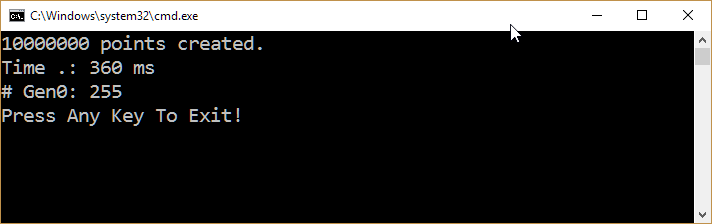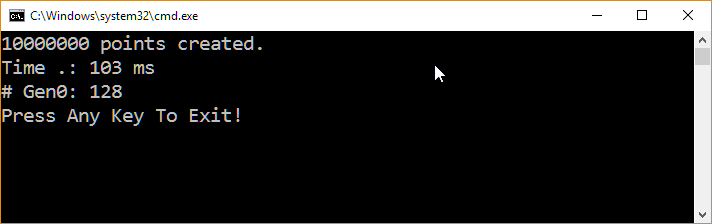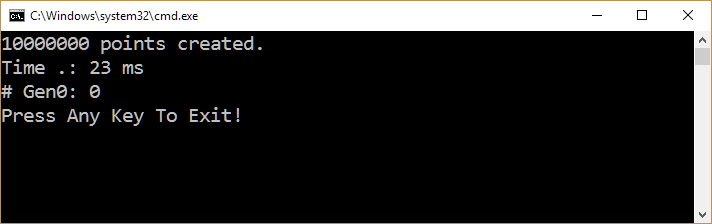In this post, let’s talk about how to implement Value Types correctly and improve the performance of your applications.

The examples are adapted from the book Pro .NET Performance. I tried to make it more realistic considering that I spent almost 20 years writing Point3 types.

## Class or Struct?

Whenever you create a new type, you have two options:

1. Create a Reference Type (class)
• Provides a richer set of features like inheritance, can be used as lock object and more.
• Easily referenced by multiple variables;
• Reference Equality.
• Allocated on the heap
• garbage-collected.
• Elements of arrays of reference types are just references to instances of the reference type residing on the heap.
• better for bigger objects
2. Create a Value Type (struct)
• Fewer features (no inheritance, no work as lock objects).
• Structural Equality
• Allocated either on the stack or inline in containing types
• Deallocated when the stack unwinds or when their containing type gets deallocated
• Elements of arrays of value types are \$the actual instances of the value type.
• In a majority of cases, value type arrays exhibit much better locality of reference (caching on CPU is better).
• should be implemented as immutable (there are exceptions)
• instances should be small objects

There are pros and cons to both options. Usually, you will choose Value Types, which are less powerful, to save memory and get better performance.

## How much improvement will you have using Value Types wisely?

It depends! 🙂

Consider the following program:

```using System;
using System.Collections.Generic;

namespace MyApp
{
class Program
{
static void Main(string[] args)
{
const int numberOfPoints = 10_000_000;

var points = new List(numberOfPoints);
for (var i = 0; i < numberOfPoints; i++)
{
{
X = i, Y = i, Z = i
});
}

Console.WriteLine(\$"{points.Count} points created.");
Console.WriteLine("Press Any Key To Exit!");
}
}

public class Point3
{
public double X;
public double Y;
public double Z;
}
}
```

On my machine, this program allocates ~430MB of RAM. Same code, just rewriting  Point3  as a struct allocates ~231MB. Almost 200MB saved! Not bad at all.

## The need for a better Equals implementation

With a list of 10,000,000 points, let’s try to find a non-existent point

```var before = GC.CollectionCount(0);
var pointToFind = new Point3{ X = -1, Y = -1, Z = -1};

var sw = Stopwatch.StartNew();
var contains = points.Contains(pointToFind);
sw.Stop();

Console.WriteLine(\$"Time .: {sw.ElapsedMilliseconds} ms");
Console.WriteLine(\$"# Gen0: {GC.CollectionCount(0) - before}");
```

This is the output on my machine:It’s not bad (we are trying to find a point in a 10000000 elements array). But…

Under the covers, List<T> uses the Equals method to compare objects. Here is the current implementation of ValueType.

```public override bool Equals(Object obj)
{
if (null == obj)
{
return false;
}
RuntimeType thisType = (RuntimeType)this.GetType();
RuntimeType thatType = (RuntimeType)obj.GetType();

if (thatType != thisType)
{
return false;
}

Object thisObj = (Object)this;
Object thisResult, thatResult;

// if there are no GC references in this object we can avoid reflection
// and do a fast memcmp
if (CanCompareBits(this))
return FastEqualsCheck(thisObj, obj);

FieldInfo[] thisFields = thisType.GetFields(BindingFlags.Instance | BindingFlags.Public | BindingFlags.NonPublic);

for (int i = 0; i < thisFields.Length; i++)
{
thisResult = ((RtFieldInfo)thisFields[i]).UnsafeGetValue(thisObj);
thatResult = ((RtFieldInfo)thisFields[i]).UnsafeGetValue(obj);

if (thisResult == null)
{
if (thatResult != null)
return false;
}
else
if (!thisResult.Equals(thatResult))
{
return false;
}
}

return true;
}
```

The generic implementation is far from the most optimized possible (Did you see the reflection thing?!)

Let’s try to make it better.

```public struct Point3
{
public double X;
public double Y;
public double Z;

public override bool Equals(object obj)
{
if (!(obj is Point3)) return false;
var other = (Point3)obj;
return
Math.Abs(X - other.X) < 0.0001 &&
Math.Abs(Y - other.Y) < 0.0001 &&
Math.Abs(Z - other.Z) < 0.0001;
}
}
```

This is the output on my machine:Much, much better!

## Running away from boxing

Did you see we had more than 100 gen0 garbage collections? Why? Like you know, value types are not stored in the heap. So why this pressure?

The Equals function, by default, expects a reference type as the parameter. Whenever you pass a value type, .NET will need to move your object to the heap (boxing it)

In our example, we are comparing a value type with 10_000_000 instances. So 10_000_000 instances will get boxed. How to prevent it? We need to implement the IEquatable interface.

```public struct Point3 : IEquatable<Point3>
{
public double X;
public double Y;
public double Z;

public override bool Equals(object obj)
{
if (!(obj is Point3 other))
{
return false;
}

return
Math.Abs(X - other.X) < 0.0001 &&
Math.Abs(Y - other.Y) < 0.0001 &&
Math.Abs(Z - other.Z) < 0.0001;
}

public bool Equals(Point3 other) =>
Math.Abs(X - other.X) < 0.0001 &&
Math.Abs(Y - other.Y) < 0.0001 &&
Math.Abs(Z - other.Z) < 0.0001;

public static bool operator ==(Point3 a, Point3 b) => a.Equals(b);

public static bool operator !=(Point3 a, Point3 b) => !a.Equals(b);
}
```

The output on my machine:No GC!

## Thinking a better GetHashCode implementation

If you use your objects as dictionary keys, consider spending some time learning about how to write a better GetHashCode version. This StackOverflow thread is a good starting point.

## Time To Action

Performance is a feature! Using Value Types can help you to improve the performance of your application dramatically. So, if everytime you create a new type, you just start writing “public class” stop right now!

PS: I spent almost 20 years of my life writing CAD systems. I have no idea how many times I wrote “Point3” types. Now, my dirty little secret: for a long time, I did it creating Point3 as a class. You are not alone!